Study Guide

# Unit Rate

## Unit Rate

A rate is a ratio of two numbers measured in different units. A unit rate is a rate that's been simplified so that one of the numbers of units is 1.

Unit rates make life easier. For example, if a cop pulls us over and says we were going 240 miles for every 3 hours, we'll probably look at her like she's crazy. But if she says our speed was 80 miles per hour instead, it's much clearer. That's 80 miles in one hour, which is a unit rate.

Having a knack for unit rates can also make us smarter shoppers. For example, let's say that we're craving some cheesy, crunchy goodness and are trying to decide between two bags of chips. The Shmoritos are \$ \$3.84 for 12 oz. The Shmingles are \$2.38 for 7 oz. We obviously want the most munch for our money, so which bag should we buy?

To solve this crunchy conundrum and find the better deal, we need to compare the price per ounce for each bag. We can write a ratio of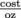for each bag, and then simplify them so that each ratio has 1 oz in the denominator. These unit rates will tell us the price for each single ounce of chips.

For the Shmoritos, the ratio of cost to ounces is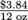. We want the denominator to be 1, so we divide both top and bottom by 12.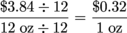There we go. The Shmoritos cost 32 cents for each ounce.

For the Shmingles, the ratio of cost to ounces is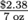. Divide both top and bottom by 7 to get rid of that 7 in the denominator.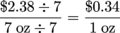So each ounce of Shmingles costs 34 cents.

Shmoritos is definitely the better buy, and it has that cheesy, crunchy goodness we've been craving. Now what to drink?